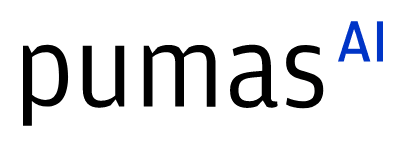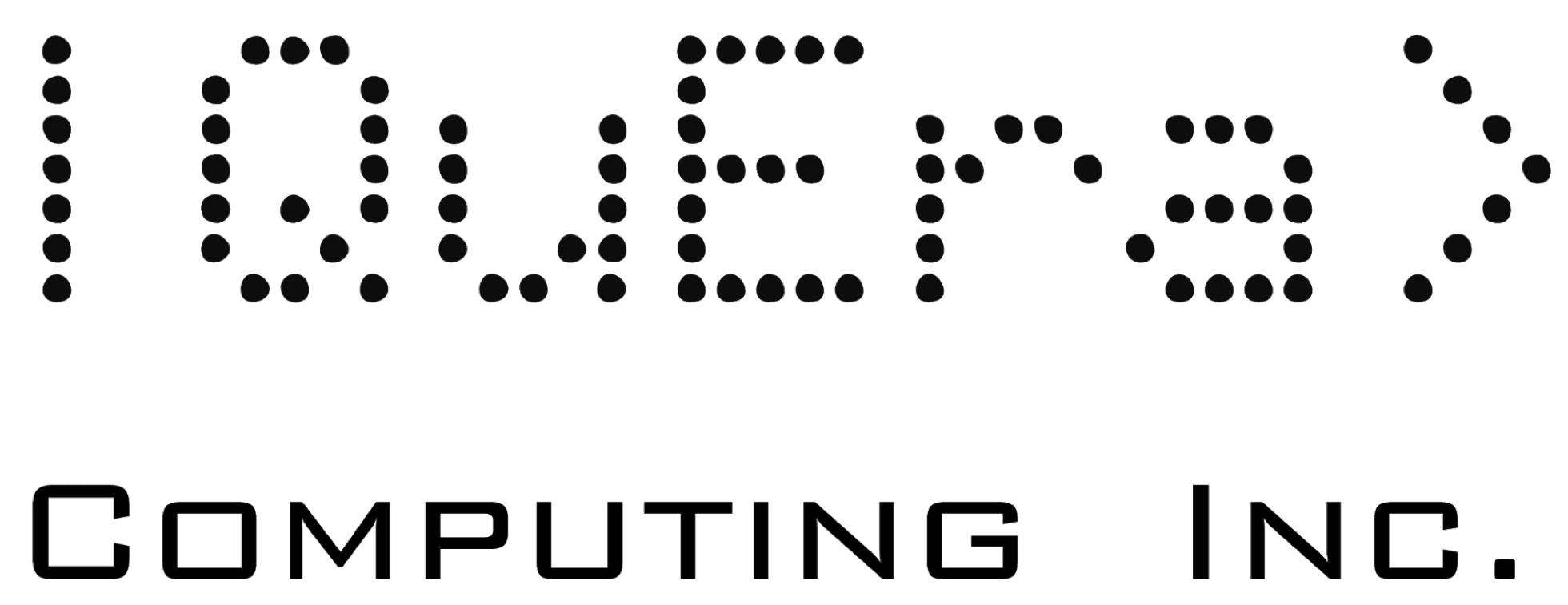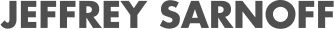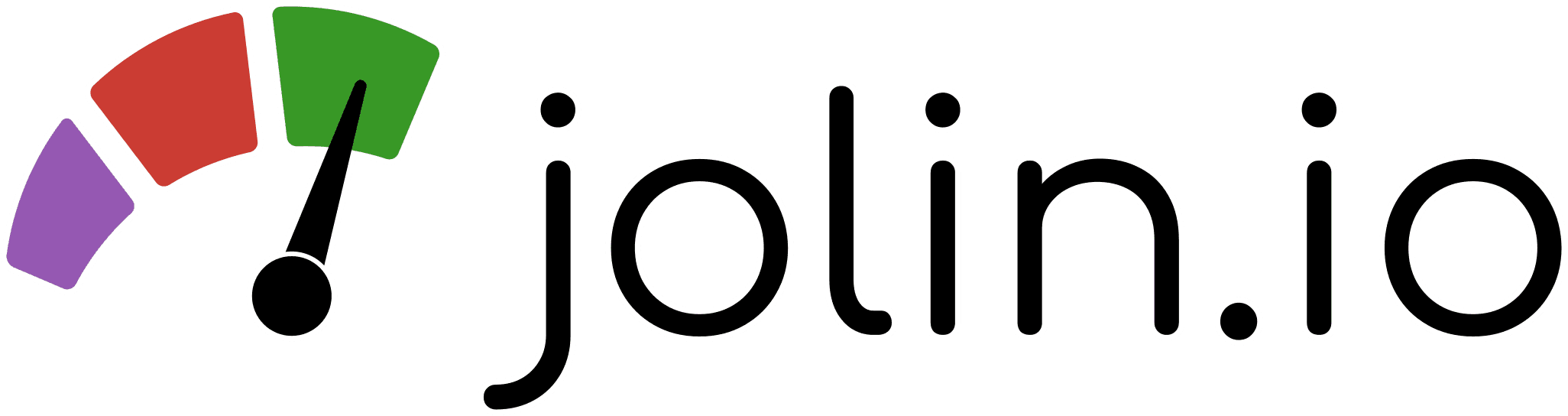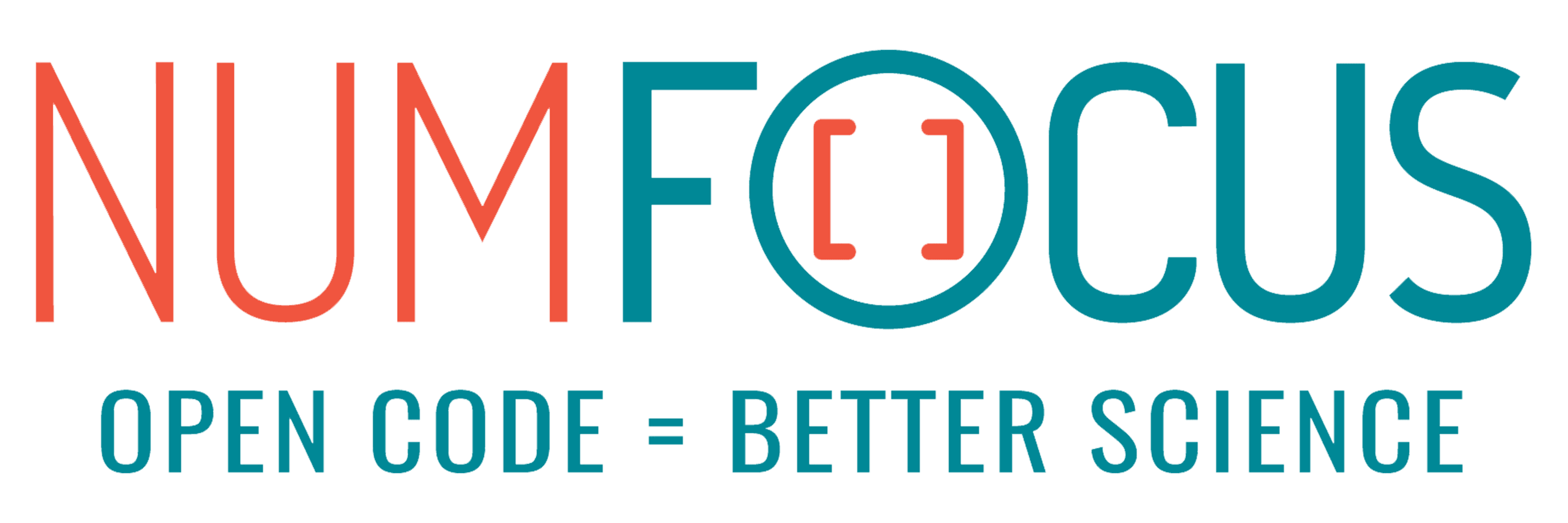# What we learned building a modelling language for macroeconomics

07/28/2023, 3:40 PM3:50 PM UTC
32-124

#### Abstract:

When we set out to build the modelling language for StateSpaceEcon.jl, our package for macroeconomic models, we did not yet know that Julia was the perfect language for the task. When faced with hundreds of variables, shocks, equations, parameters, lags and expectation terms, what the economist needs most is an intuitive and expressive domain-specific language to help keep all that complexity under control. Join us as we share our experience – it might help you enhance your own packages.

#### Description:

StateSpaceEcon.jl is designed to work with discrete-time macroeconomic state space models. These models typically include from tens to hundreds of variables, equations and parameters. Variables come in several different kinds (exogenous, endogenous, observed, shocks, etc.), parameters are often linked to other parameters, and equations can be linear or non-linear containing past and expected future values of the variables as well as mathematical expressions (arithmetic operations, powers, exponents, logarithms, etc.) and time-series operations (e.g., moving averages or weighted sums). In order to keep the complexity of expressing the model separate from that of working with it, we have designed and implemented a modelling language that supports the necessary features.

In this presentation, we would like to share with our audience how we designed and implemented the domain-specific language for StateSpaceEcon.jl and what we learned along the way. With concrete and practical examples, we will explain the motivations behind our design decisions and demonstrate a few key techniques we employed in our implementations. Specifically, we plan to cover the following aspects.

1. How the model developer can declare the various properties of the model, namely variables, shocks, parameters and equations.
2. Explain why we encapsulate each model into its own Julia module.
3. How we manipulate model equations to automatically compute the dynamic and steady state residuals and their derivatives.
4. How we implement the ability to express time series operations in the model equations, such as moving averages, and how we allow for user-defined extensions.
5. How we deal with parameters that are functions of other parameters.

If time permits, we can also discuss the following. 6. How we allow additional constraints for the steady state system of the model. 7. How we assemble the residual functions into a stacked-time global system and how we construct its sparse Jacobian matrix. 8. How we linearize individual equations or the entire system. 9. Our discrete time series type descendent from AbstractVector, together with its primitive frequency type.